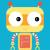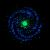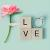## 17.输入一个数字如２０３８０９０８判断这个数字所代表的日期是这个年的第几个星期（这一年从1月1日算起）

0
SimepleDateFormat将字符串转成日期，再用w占位符，格式化出这个数字即可。
0```package com.ea.test;

import java.util.HashMap;
import java.util.Map;
import junit.framework.TestCase;
import org.junit.Test;
import com.ea.entity.CacheConfig;

public class OtherTest extends TestCase{

private static String targetDate = "20380908"; //声明一个日期字符串

/*
* 初始化日期，将年、月、日分别以year、month、day的键存取
*/
private static Map<String,String> initDate(){
Map<String,String> initResult = new HashMap<String,String>();
String year = targetDate.substring(0,4);
String month = targetDate.substring(4,6);
String day = targetDate.substring(6);

initResult.put("year", year);
initResult.put("month",month);
initResult.put("day", day);

return initResult;
}

/*
* 判断是否是闰年
*/
private static boolean isRuenYear(){
Map<String,String> initResult = initDate();
int year = Integer.parseInt( initResult.get("year") );

return (((year % 4 == 0) && (year % 100 != 0)) || year % 400 == 0) ? true : false;
}

/*
* 判断该日是该年的第多少天
*/
private static int dayNumber(){
//30天跟31天的月数开始初始化都为0
int month_30 = 0;
int month_31 = 0;

int month = Integer.parseInt( initDate().get("month") );
for(int i = 1; i < month; i++){
if(i == 4 || i == 6 || i == 11)
month_30++;
else
month_31++;
}

int result = month_30 * 30 + month_31 * 31;
return isRuenYear() ? result - 3 : result - 2;
}

@Test
public void testFinal(){
int dayNumber = OtherTest.dayNumber();
int zhouNumber = dayNumber % 7 == 0 ? dayNumber / 7 : dayNumber / 7 + 1;
System.out.println(targetDate + " 是这年的第 " + zhouNumber + " 个星期!");
}

}```

0`System.out.println(new SimpleDateFormat("w").format(new SimpleDateFormat("yyyyMMdd").parse("20380908")));`
0```import java.text.ParseException;
import java.text.SimpleDateFormat;
import java.util.Date;

public class DateTest
{

private static String getWeekNum(String targetDate) throws ParseException
{
SimpleDateFormat sdf = new SimpleDateFormat("yyyyMMdd");
//将目标时间转换成date
Date date = sdf.parse(targetDate);
//将date格式化输出，w指代一年中的星期数
SimpleDateFormat sdf2 = new SimpleDateFormat("w");
return sdf2.format(date);
}

public static void main(String[] args) throws ParseException
{
String targetDate = "20380908";
System.out.println(targetDate+"是这年的第"+getWeekNum(targetDate)+"个星期");
}
}```